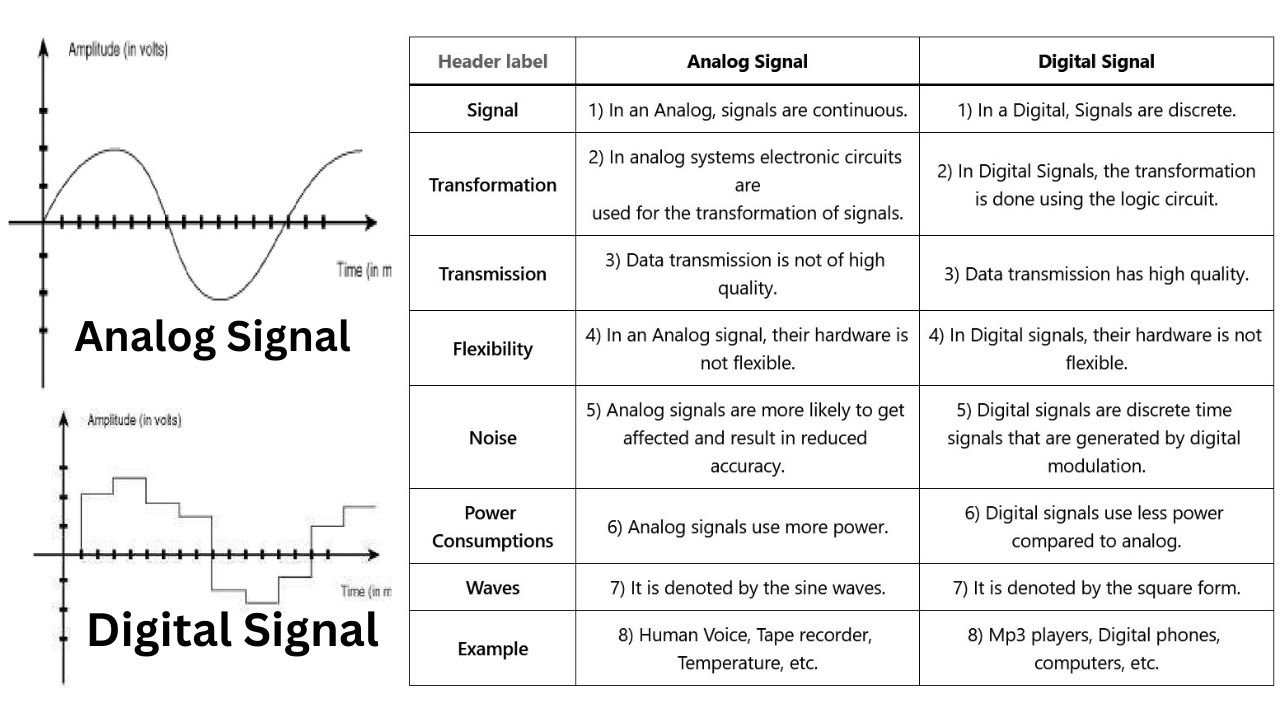# What are Analog and Digital Signals in a Computer Network?Before knowing analog and digital signals, first, let’s see what is signal?

## What is Signal?

A Signal is an electromagnetic wave that is used to communicate system-to-system by sending data from one network to another network is basically known as “Signal”.

In a computer network there are mainly two types of signals are:

1. Analog Signal
2. Digital Signal

## What is Analog Signal?

An Analog signal is a signal which is continuous and has a time-varying feature. It is a representation of time-varying quantity. For example, the Human voice can be considered an analog signal because the signal of the human voice flows in a continuous manner.

In other words, we can say that the analog signal is represented by the continuous variable which transmits the information/data as a response to physical phenomenon. It is known as an “Analog Signal”

Examples of digital signals are Temperature, Pressure, Flow Measurement, etc.

### Types of Analog Signal

1. Simple Analog Signal
2. Composite Analog Signal

## What is Digital Signal?

As the word suggests “Digital” which means it describes the electronic technology that generates signals. It is a physical signal that is represented by two discrete values “0” & “1”, these discrete values are known as bitstream.

(If you want to know more about these discrete values then you should read our this article: What is the difference Between Bit and Byte?

In simple words, we can say that the binary signals are known as “Digital signals” where the signals are converted into a small bit form which is represented by a series of “0” & “1”.